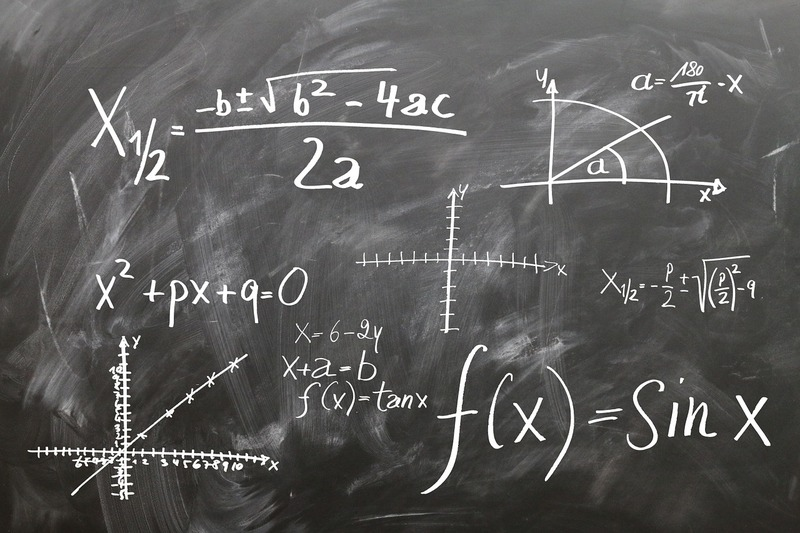If you’re anything like me, I am much more reliant on my calculator than I’d like to admit. I often do even the simplest calculations like adding whole numbers (no, not even multiple digits) using a calculator. So the idea of a NO CALCULATOR SAT Math section sounded daunting.

Thankfully, it’s not as daunting as it sounds. First of all, there’s only one math section on the SAT that does not allow a calculator. It is also the shortest section, timing in at 25 minutes, and is always the third section.

Follow these tips below to master the No Calculator SAT Math:

#### 1. Find the simple solution

If the calculation or answer is too complicated, it’s probably wrong. Since math problems in this section are intended to be done without a calculator, if you find yourself writing out a string of digits after the decimal or doing a hundred step complex math problem, you should stop and consider whether or not your initial solution is correct. Additionally, a key component to the SAT Math is testing basic underlying concepts and oftentimes there is an elegant solution to the problem. If you are able to analyze the problem effectively and understand what core concepts each problem is identifying, it’ll help tremendously in solving the equations.

#### 2. Review math concepts

Make sure you have a solid grasp on arithmetic, algebra, linear equations, geometry, basic trigonometry, and complex numbers. For a complete detailed list, check out the College Board site here. If you find yourself needing more help reviewing these concepts, sign-up for one-on-one tutoring with one of our excellent UC Berkeley tutors.

#### 3. Do lots of practice problems

And by practice I don’t mean just a little. Practice as many questions as possible. This will not only help you get faster, but help you identify and zero in on the type of problems you struggle with most.

#### 4. Write out the problems

It’s tempting to try to do all the steps in your head or do shortcuts. Don’t! This is an easy way to make simple mistakes and lose track of where you are. Writing down your problem-solving will also make it easier to revisit the problem again later.

#### 5. Practice mental Math

Of course, no calculator means that you will have to do a lot of mental math. Make sure you are able to do simple calculations quickly and effortlessly.

Accomplish this and you’ll be well on your way to ace-ing the SAT Math, sans calculator.

Do you have any tips for the no calculator section? Share in the comments below!